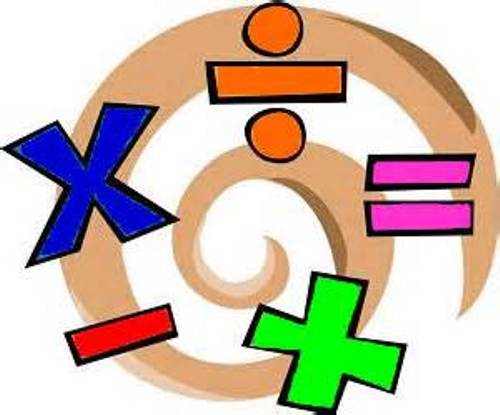# Calculus

Teacher:Kerrie Pratt
Extra Help:Thursday 3-4 PM, Rm B45 or by appointment

Course Summary

In this course students will use the tools they have developed and practiced in Algebra, Geometry, Precalculus and Trigonometry to expand their abilities to gather information and make predictions about functions.

During the first semester, students will explore limits, the tangent line problem and the derivative of a function. In addition students will study the relationship between differentiability and continuity.

In the second semester, students will use their understanding of the derivative to explore the integral of a function. Students will use a variety of basic integration rules to find antiderivatives as well as finding the area under a curve using various methods.

Throughout the year, students will be applying the techniques they acquire to solve and analyze real world problems.

Essential Questions:

• In what ways do we think mathematically when problem solving?

• How do we determine the best approaches to solving a problem?

• How can we use mathematics to describe events that occur in the world?

• How do we summarize and communicate conclusions mathematically?

Schedule of Major Topics

Semester 1

September            Limits and Their Properties
October                Tangent Problem
November            Differentiation
December            Applications of Derivation/Related Rates of Change

Semester 2

January                 Summations/Approximating Area
February               Basic Rules of Integration
March                   Area under the Curve
April                     Exponential and Log Functions with Calculus
May                      Trig Functions with Calculus
June                      Wrap Up

*Students can expect to complete a project for each unit we study.